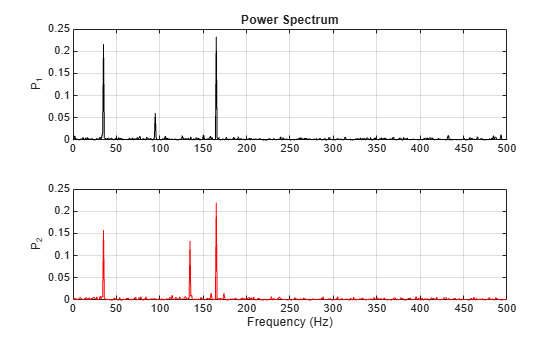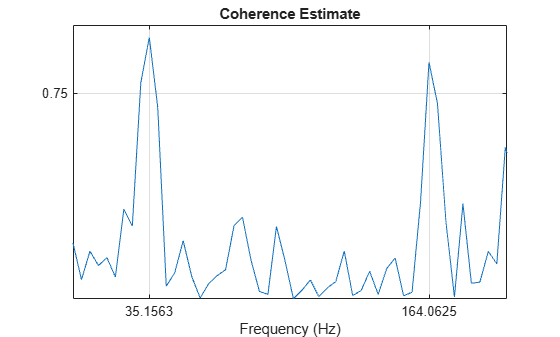# Compare the Frequency Content of Two Signals

Spectral coherence helps identify similarity between signals in the frequency domain. Large values indicate frequency components common to the signals.

Load two sound signals into the workspace. They are sampled at 1 kHz. Compute their power spectra using `periodogram` and plot them next to each other.

```load relatedsig Fs = FsSig; [P1,f1] = periodogram(sig1,[],[],Fs,'power'); [P2,f2] = periodogram(sig2,[],[],Fs,'power'); subplot(2,1,1) plot(f1,P1,'k') grid ylabel('P_1') title('Power Spectrum') subplot(2,1,2) plot(f2,P2,'r') grid ylabel('P_2') xlabel('Frequency (Hz)')```Each signal has three frequency components with significant energy. Two of those components appear to be shared. Find the corresponding frequencies using `findpeaks`.

```[pk1,lc1] = findpeaks(P1,'SortStr','descend','NPeaks',3); P1peakFreqs = f1(lc1)```
```P1peakFreqs = 3×1 165.0391 35.1562 94.7266 ```
```[pk2,lc2] = findpeaks(P2,'SortStr','descend','NPeaks',3); P2peakFreqs = f2(lc2)```
```P2peakFreqs = 3×1 165.0391 35.1562 134.7656 ```

The common components are located around 165 and 35 Hz. You can use `mscohere` to find the matching frequencies directly. Plot the coherence estimate. Find the peaks above a threshold of 0.75.

```[Cxy,f] = mscohere(sig1,sig2,[],[],[],Fs); thresh = 0.75; [pks,locs] = findpeaks(Cxy,'MinPeakHeight',thresh); MatchingFreqs = f(locs)```
```MatchingFreqs = 2×1 35.1562 164.0625 ```
```figure plot(f,Cxy) ax = gca; grid xlabel('Frequency (Hz)') title('Coherence Estimate') ax.XTick = MatchingFreqs; ax.YTick = thresh; axis([0 200 0 1])```You get the same values as before. You can find the frequency content common to two signals without studying the two signals separately.